In this section: How to:Specify Color Properties

Color properties define the color of an object. All objects can be assigned a color and transparency setting. Area and line objects can be assigned a color definition or a gradient definition.

 Top of page
Syntax: How to Specify Color Properties
`color: 'string'`

where:

color: string

Can be one of the following:

• A color name (for example, 'red').

For a list of supported color names, see

• Three RGB values, or three RGB values and a transparency setting:
`'rgb (r,g,b)'`

or

`'rgba(r,g,b,a)')`

The values r, g, and b represent the intensity (from 0 to 255) of red, green, and blue.

Transparency defines how the object blends into the background, expressed as a number between 0.0 (fully transparent) and 1.0 (fully opaque).

For example, the color black can be described as 'rgb(0,0,0)'

• Three Hue-Saturation-Lightness (HSL) values, or three HSL values and a transparency setting:
`'hsl(h,s,l)'`

or

`'hsla(h,s,l,a)'`
• Hue is expressed as an angle on the color wheel. Red is at the top and is defined as 0 or 360°, green is 120°, and blue is 240°.
• Saturation defines how pure the hue is, as a percentage, including a percent symbol (%). A pure color is 100% saturated, while grays are unsaturated.
• Lightness defines how light or dark the hue is, as a percentage, including a percent symbol. White is 100% lightness, while black is 0% lightness.
• Transparency defines how the object blends into the background, expressed as a number between 0.0 (fully transparent) and 1.0 (fully opaque).
`'#hexvalue'`

The hexadecimal color value starts with a pound sign (#), then has two hexadecimal digits each for the combination of red, green, and blue color values (RGB). The lowest value for each component is 0 (hex 00). The highest value is 255 (hex FF).

For example, black is #000000, which corresponds to rgb(0,0,0) . Red is #FF0000, which corresponds to rgb(255,0,0). White is #FFFFFF, which corresponds to rgb(255,255,255).

The World Wide Web Consortium (W3C) website at http://www.w3.org/TR/css3-color/#colorunits provides details about color specifications.

 Top of page
Example: Defining Colors

The following request has five series. The JSON definitions for each series illustrate one type of color definition, and display that color definition in the legend as the series label. The heading and border also have color definitions:

```GRAPH FILE WF_RETAIL_LITE
"Color: RGB(0 142 126)"
SUM COGS_US GROSS_PROFIT_US REVENUE_US DISCOUNT_US MSRP_US
WHERE COUNTRY_NAME EQ 'United States'
ON GRAPH PCHOLD FORMAT JSCHART
ON GRAPH SET LOOKGRAPH VBAR
ON GRAPH SET STYLE *
*GRAPH_JS
legend: {visible: true},
border: {width: 1, color: 'navy'},
series: [
{series: 0, color: 'rgb(0,142,126)', label: 'rgb(0,142,126)'},
{series: 1, color: 'hsla(240, 100%, 50%, 0.1)',
label:'hsla(240, 100%, 50%, 0.1)'},
{series: 2, color: 'hsl(240, 100%, 50%)',label:'hsl(240, 100%, 50%)'},
{series: 3, color: '#00FF00',label:'#00FF00'},
{series: 4, color: 'cyan',label: 'cyan'}
]
*END
TYPE=HEADING, COLOR = rgb(0 142 126), JUSTIFY=LEFT,\$
ENDSTYLE
END```

The output is: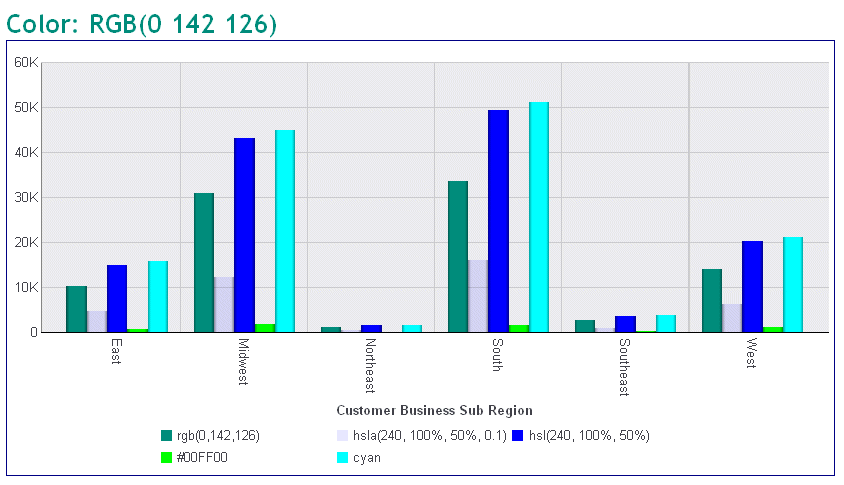Top of page
 How to:

Gradients are transitions between colors. The transitions can be applied linearly across the chart or radially from a central point outward.

Gradients can be defined as a string or a JSON object.

 Top of page
Syntax: How to Define Linear Gradient Properties as a String
```color: 'linear-gradient(startX, startY, endX, endY,
stopsOffset  stopsColor,...stopsOffset  stopsColor)'```

where:

startX, starty

Is the starting x-axis and y-axis position for the color gradient.

The axis positions can be specified as numbers (for example, 0) or as strings (for example, '5%'). Numbers are interpreted as raw Scalable Vector Graphics (SVG) pixel coordinates. For example, start: x:0, y:0 is the top-left corner of the object. Negative numbers are not valid. Any number less than zero is treated as zero. Numeric start and end coordinates are only useful for objects where you can set the area dimensions (such as the chart background area). For example, if the chart background area is set to 200 by 200 pixels, and start x:0, y:0 and stop x:100, y:0 are used to define a linear gradient, the gradient will be applied from the left edge to the center of the rectangle. If the size of the object is calculated by the library (for example, legend area, chart frame, risers), string percentages are typically used.

endX, endy

Applies to linear gradients only. Is the ending x-axis and y-axis position for the color gradient.

The axis positions can be specified as numbers (for example, 20) or as strings (for example, '20%'). Numbers are interpreted as raw Scalable Vector Graphics (SVG) pixel coordinates. For example, start: x:0, y:0 is the top-left corner of the object. Negative numbers are not valid. Any number less than zero is treated as zero. Numeric start and end coordinates are only useful for objects where you can set the area dimensions (such as the chart background area). For example, if the chart background area is set to 200 by 200 pixels, and start x:0, y:0 and stop x:100, y:0 are used to define a linear gradient, the gradient will be applied from the left edge to the center of the rectangle. If the size of the object is calculated by the library (for example, legend area, chart frame, risers), string percentages are typically used.

stopsOffset

Offset values define where the color changes. They can be numbers, or they can be strings enclosed in single quotation marks with a percent symbol (%). Only numbers between 0 and 1 are valid, and are treated as percentages. A value 0.5 is the same as 50%. Numbers less than zero are treated as zero, and numbers greater than one are treated as 1.

stopsColor

Specifies a color for the corresponding offset.

 Top of page

The following request specifies a linear gradient for the background of the chart. The start and end coordinates describe the entire chart area, and the gradient transitions from teal to cyan. The linear gradient is specified as a string:

```GRAPH FILE WF_RETAIL_LITE
SUM COGS_US
ACROSS PRODUCT_SUBCATEG
ON GRAPH PCHOLD FORMAT JSCHART
ON GRAPH SET LOOKGRAPH LINE
ON GRAPH SET STYLE *
*GRAPH_JS
fill: {
color: 'linear-gradient(0,0,100%,100%, 20% teal, 95% cyan)'
},
series: [{series:0, color: 'black'}]
*END
ENDSTYLE
END```

The output is: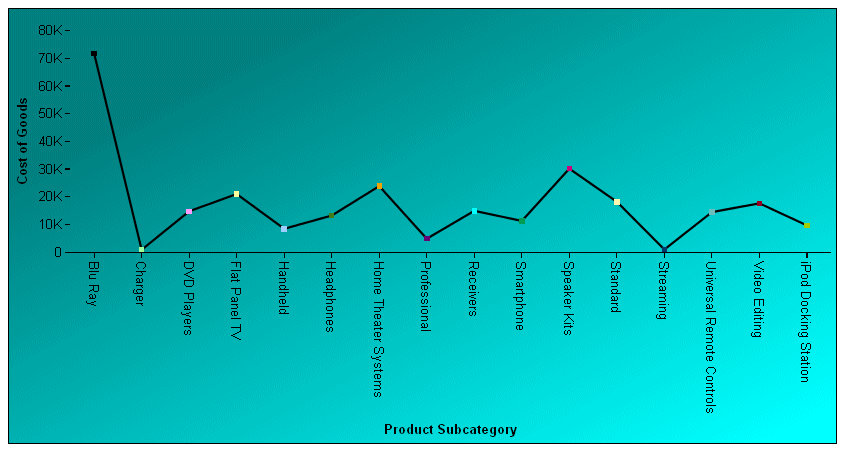Top of page
```color: 'radial-gradient(startX, startY, radius,
stopsOffset, stopsColor,...stopsOffset stopsColor)'```

where:

startX, starty

Is the starting x-axis and y-axis position for the color gradient.

The axis positions can be specified as numbers (for example, 0) or as strings (for example, '5%'). Numbers are interpreted as raw Scalable Vector Graphics (SVG) pixel coordinates. Numeric start and end coordinates are only useful for objects where you can set the area dimensions (such as the chart background area). If the size of the object is calculated by the library (for example, legend area, chart frame, risers), string percentages are typically used. For radial gradients, start: x/y defines the center of the gradient (for example, start: x: '50%', y: '50%' draws the gradient starting from the center of the object).

stopsOffset

Offset values define where the color changes. They can be numbers, or they can be strings with a percent symbol (%). Only numbers between 0 and 1 are valid, and are treated as percentages. A value 0.5 is the same as 50%. Numbers less than zero are treated as zero, and numbers greater than one are treated as 1.

stopsColor

Specifies a color for the corresponding offset.

 Top of page

The following request specifies a radial gradient for the background of the chart. The start position and radius describe the entire chart background. From inside to outside, the colors transition from blue to red to blue to red to lightblue. The gradient is specified as a string:

```GRAPH FILE WF_RETAIL_LITE
SUM AVE.COGS_US MDN.COGS_US
BY PRODUCT_CATEGORY
ON GRAPH PCHOLD FORMAT JSCHART
ON GRAPH SET STYLE *
*GRAPH_JS
fill: {color:
}
*END
ENDSTYLE
END```

The output is: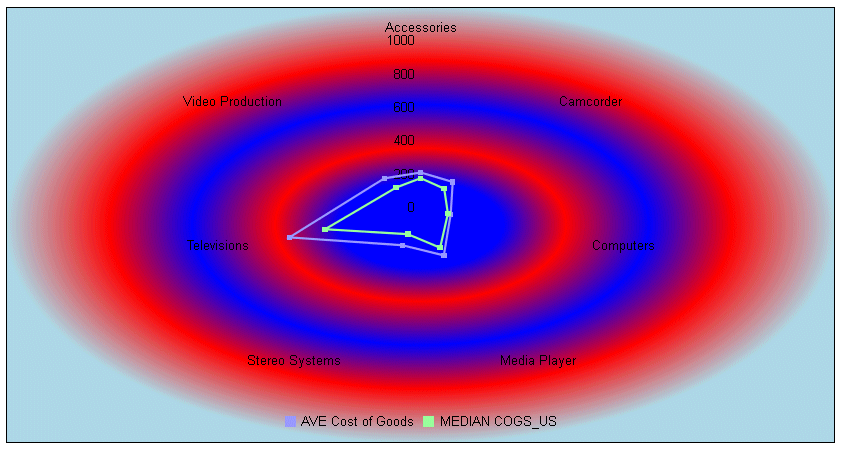Top of page
Syntax: How to Define Gradient Properties as a JSON Object
```color: {
type: 'string',
start:{
x: x,
y: y   },
end:{
x: x,
y: y   },
stops: [
{
offset: offset,
color: 'string'
},
...
]
}```

where:

'string'

Is a string that defines the type of gradient. Valid values are 'linear' or 'radial'.

start: x, start: y,

Specify the gradient coordinates in the chart.

x: x

Specifies the starting x-coordinate in the chart. It can be specified as a number or as a percent value enclosed in single quotation marks and including the percent symbol.

Numbers are interpreted as raw Scalable Vector Graphics (SVG) pixel coordinates. For example, start: x:0, y:0 is the top-left corner of the object. Negative numbers are not valid. Any number less than zero is treated as zero.

Percentage coordinates are most useful when the size of an object is calculated by the library or, for radial gradients, where 50% specifies the center of the object.

y: y

Specifies the starting y-coordinate in the chart. It can be specified as a number or as a percent value enclosed in single quotation marks and including the percent symbol.

Numbers are interpreted as raw Scalable Vector Graphics (SVG) pixel coordinates. For example, start: x:0, y:0 is the top-left corner of the object. Negative numbers are not valid. Any number less than zero is treated as zero.

Percentage coordinates are most useful when the size of an object is calculated by the library or, for radial gradients, where 50% specifies the center of the object.

end: x, end: y

Specify the gradient coordinates in the chart.

x: x

Applies to linear gradients only. Specifies the ending x-coordinate in the chart. It can be specified as a number or as a percent value enclosed in single quotation marks and including the percent symbol.

Numbers are interpreted as raw Scalable Vector Graphics (SVG) pixel coordinates. Negative numbers are not valid. Any number less than zero is treated as zero.

Percentage coordinates are most useful when the size of an object is calculated by the library or for radial gradients, where 50% specifies the center of the object.

y: y

Applies to linear gradients only. Specifies the ending y-coordinate in the chart. It can be specified as a number or as a percent value enclosed in single quotation marks and including the percent symbol.

Numbers are interpreted as raw Scalable Vector Graphics (SVG) pixel coordinates. Negative numbers are not valid. Any number less than zero is treated as zero.

Percentage coordinates are most useful when the size of an object is calculated by the library or for radial gradients, where 50% specifies the center of the object.

The start: x/y and end: x/y parameters can be specified as numbers (for example, 0/20) or as strings (for example, '5%'/'20%'). Numbers are interpreted as raw Scalable Vector Graphics (SVG) pixel coordinates. For example, start: x:0/y:0 is the top-left corner of the object. Negative numbers are not valid. Any number less than zero is treated as zero. Numeric start/end coordinates are only useful for objects where you can set the area dimensions (such as the chart background area). For example, if the chart background/draw area is set to 200 by 200 pixels and start x:0/y:0 and stop x:100/y:0 is used to define a linear gradient, the gradient will be applied from the left edge to the center of the rectangle. If the size of an object is calculated by the library (such as for the legend area, chart frame, or risers), string percentages are typically used. For radial gradients, start: x/y defines the center of the gradient (for example, start: x: '50%'/y: '50%' draws the gradient in the center of the object).

Applies to radial gradients only. Defines the distance from the center to the outermost edge of the gradient.

For example, if an object is 200 by 200 pixels with a gradient start: x:'50%', y:'50%' and radius: '100%', the outermost gradient edge will be 100 pixels beyond the edges of the object. In this example, 100% of 200 pixels is 200 pixels, so the gradient is actually 400 pixels from extreme left to extreme right. For a radial gradient that starts in the center (start: x:'50%', y:'50%'), radius: '50%' is normally used to draw the gradient from the center to the outermost edges of the object.

stops:

Is a comma-separated list of offset-color pairs. This array can be any length, and can be a list of objects or arrays.

offset: offset

Is an offset expressed as a number or a percent.

If expressed as a number, it must be between 0 and 1 and represents a percentage of the gradient area at which the color changes. The value 0.5 is the same as 50%. Numbers less than zero are treated as zero, and numbers greater than one are treated as 1.

If expressed as a percentage, it must be enclosed in single quotation marks and include the percent symbol. It specifies a percentage of the gradient area at which the color changes.

color: 'string'

Specifies the color for the corresponding offset.

 Top of page
Example: Sample Linear Gradient JSON Object

The following request specifies a linear gradient for the background of the chart. The start and end coordinates describe the entire chart area, and the gradient transitions from teal to cyan. The linear gradient is specified as a JSON object:

```GRAPH FILE WF_RETAIL_LITE
SUM COGS_US
ACROSS PRODUCT_SUBCATEG
ON GRAPH PCHOLD FORMAT JSCHART
ON GRAPH SET LOOKGRAPH LINE
ON GRAPH SET STYLE *
*GRAPH_JS
fill: {color:
{type: 'linear',
start: {x: 0, y: 0},
end: {x:'100%', y: '100%' },
stops: [
{offset: '20%', color: 'teal'},
{offset: '95%', color: 'cyan'}
]
}},
series: [{series:0, color: 'black'}]
*END
ENDSTYLE
END```

The output is: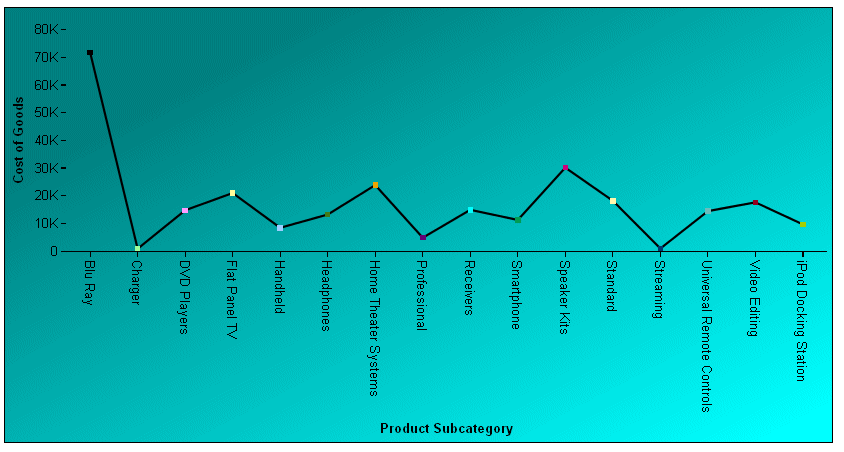Top of page

The following request specifies a radial gradient for the background of the chart. The start position and radius describe the entire chart background. From inside to outside, the colors transition from blue to red to blue to red to lightblue. The gradient is specified as a JSON object:

```GRAPH FILE WF_RETAIL_LITE
SUM AVE.COGS_US MDN.COGS_US
BY PRODUCT_CATEGORY
ON GRAPH PCHOLD FORMAT JSCHART
ON GRAPH SET STYLE *
*GRAPH_JS
fill: {
color:
start: {x: '50%', y: '50%'},
end: {x:'50%', y: '50%' },
stops: [
{offset: '20%', color: 'blue'},
{offset: '35%', color: 'red'},
{offset: '55%', color: 'blue'},
{offset: '75%', color: 'red'},
{offset: 1, color: 'lightblue'}
]
}}
*END
ENDSTYLE
END```

The output is: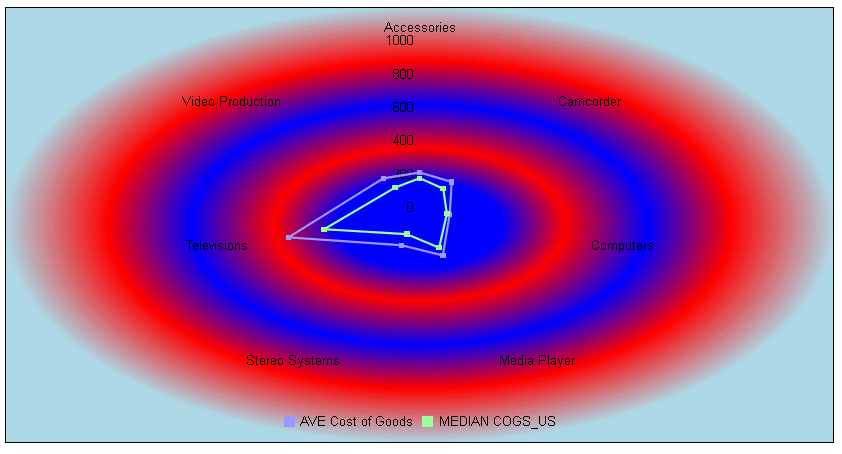Top of page
SVG Color Patterns
 How to:Define an SVG Color Pattern

In some cases, you may want to use patterns to distinguish chart elements such as risers and markers, or to fill chart frames. This can be a useful technique in black and white environments where colors are not distinguishable.

 Top of page
Syntax: How to Define an SVG Color Pattern
```color:{
type:'pattern',
color: 'string',
backgroundColor: 'string',
shape: 'string',
size: number,

where:

color: 'string'

Defines the color of the pattern shape using a color name or numeric specification string.

backgroundColor: 'string'

Defines the background color of the pattern space using a color name or numeric specification string.

shape: 'string'

Defines the shape for the pattern character. All marker shapes are supported. For information, see

size: number

Is the size, in pixels, of the pattern shape.

Is the size, in pixels, of the padding between the pattern shapes.

 Top of page
Example: Using a Pattern to Fill the Chart Frame

The following request fills the chart frame with triangles whose color is a version of blue and whose size is 10 pixels. The space between triangles is 8 pixels, and the background color is light gray:

```GRAPH FILE WF_RETAIL_LITE
SUM AVE.COGS_US MDN.COGS_US
BY PRODUCT_CATEGORY
ON GRAPH PCHOLD FORMAT JSCHART
ON GRAPH SET LOOKGRAPH VBAR
ON GRAPH SET STYLE *
*GRAPH_JS
fill: {
color:{
type:'pattern',
color: '#00aeef',
backgroundColor: 'lightgray',
shape: 'triangle',
size: 10,
*END
ENDSTYLE
END```

The output is: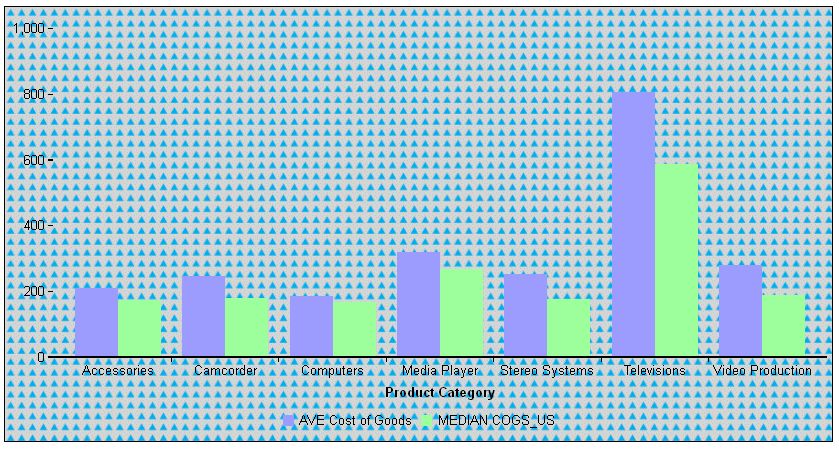Top of page
Example: Using a Pattern to Fill Series Risers

The following request defines patterns to color the risers for each series in a vertical bar chart:

```GRAPH FILE WF_RETAIL_LITE
SUM COGS_US GROSS_PROFIT_US REVENUE_US
BY PRODUCT_CATEGORY
ON GRAPH PCHOLD FORMAT JSCHART
ON GRAPH SET LOOKGRAPH VBAR
ON GRAPH SET STYLE *
*GRAPH_JS
series:[
{series:0, color:{type:'pattern',color: 'blue',backgroundColor: 'lightgray',shape: 'triangle',size: 10,pad: 8}} ,
{series:1, color:{type:'pattern',color: 'red',backgroundColor: 'beige',shape: 'plus',size: 10,pad: 8}} ,
{series:2, color:{type:'pattern',color: 'green',backgroundColor: 'yellow',shape: 'house',size: 10,pad: 8}}
]
*END
ENDSTYLE
END```

The output is: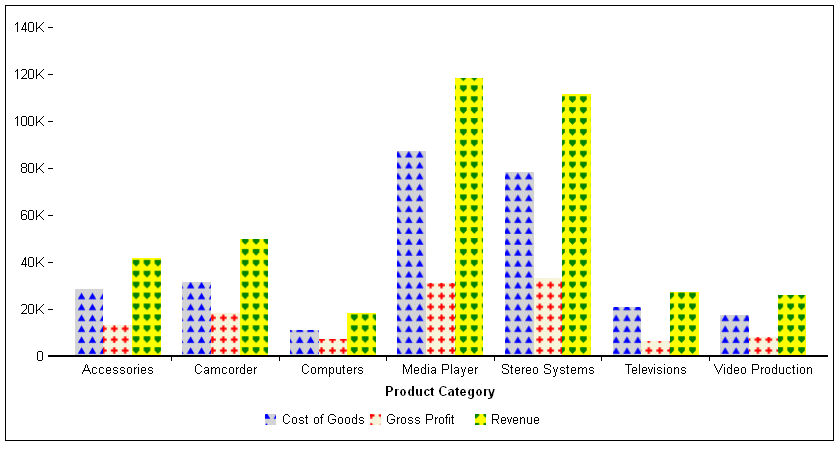Top of page
Example: Using a Pattern to Fill Pie Slices

The following request defines patterns to color the risers for each series in a pie chart:

```GRAPH FILE WF_RETAIL_LITE
SUM COGS_US
ACROSS PRODUCT_CATEGORY
ON GRAPH SET AUTOFIT ON
ON GRAPH PCHOLD FORMAT JSCHART
ON GRAPH SET LOOKGRAPH PIE
ON GRAPH SET STYLE *
*GRAPH_JS
series: [
{series:0, color:{type:'pattern',color: 'blue',backgroundColor: 'lightgray',shape: 'triangle',size: 10,pad: 8}} ,
{series:1, color:{type:'pattern',color: 'red',backgroundColor: 'beige',shape: 'plus',size: 10,pad: 8}} ,
{series:2, color:{type:'pattern',color: 'green',backgroundColor: 'yellow',shape: 'house',size: 10,pad: 8}},
{series: 3, color: {type:'pattern',color: 'orange',backgroundColor: 'antiquewhite',shape: 'square',size: 10,pad: 8}},
{series: 4, color: {type:'pattern',color: 'lightgreen',backgroundColor: 'white',shape: 'arrow',size: 10,pad: 8}},
{series: 5, color: {type:'pattern',color: 'steelblue',backgroundColor: 'cyan',shape: 'fiveStar',size: 10,pad: 8}},
{series: 6, color: {type:'pattern',color: 'slateblue',backgroundColor: 'skyblue',shape: 'sixStar',size: 10,pad: 8}}
]
*END
ENDSTYLE
END```

The output is: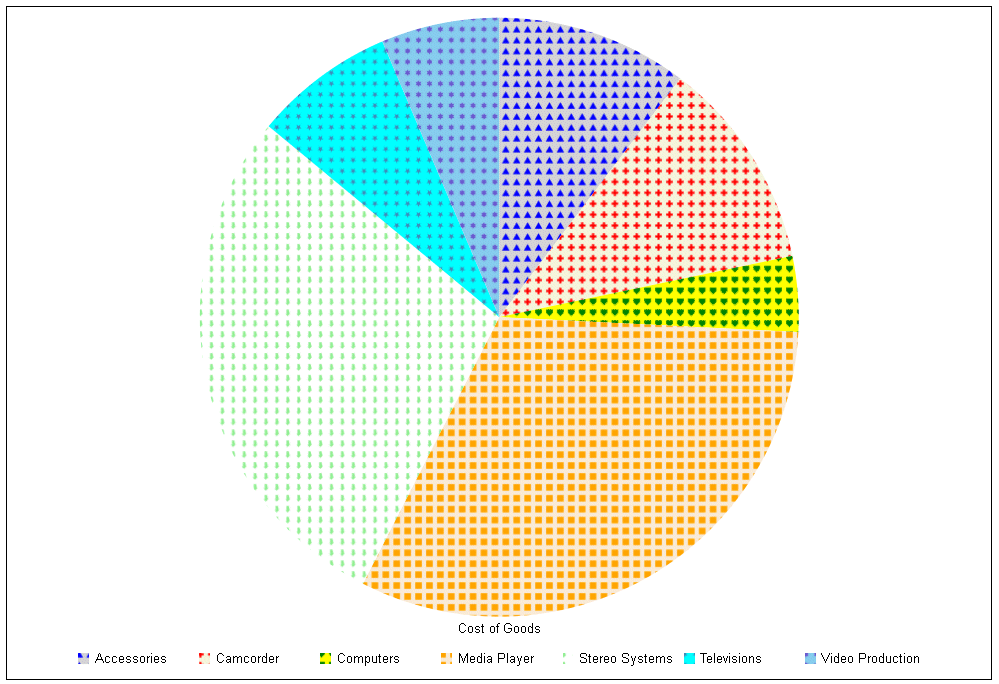Top of page
Example: Using a Pattern to Fill Series Markers

The following request generates a scatter chart. The markers for each series are filled with patterns and are sized large enough to fit the patterns:

```GRAPH FILE WF_RETAIL_LITE
SUM AVE.COGS_US GROSS_PROFIT_US MSRP_US
ACROSS PRODUCT_CATEGORY
ON GRAPH PCHOLD FORMAT JSCHART
ON GRAPH SET LOOKGRAPH SCATTERS
ON GRAPH SET STYLE *
*GRAPH_JS
series: [
{series:0, marker:{size:15},color:{type:'pattern',color: 'blue',backgroundColor: 'lightgray',shape: 'triangle',size: 10,pad: 8}},
{series:1, marker:{size:20},color:{type:'pattern',color: 'red',backgroundColor: 'beige',shape: 'plus',size: 10,pad: 8}},
{series:2, marker:{size:30},color:{type:'pattern',color: 'green',backgroundColor: 'yellow',shape: 'house',size: 10,pad: 8}}
]
*END
ENDSTYLE
END```

The output is: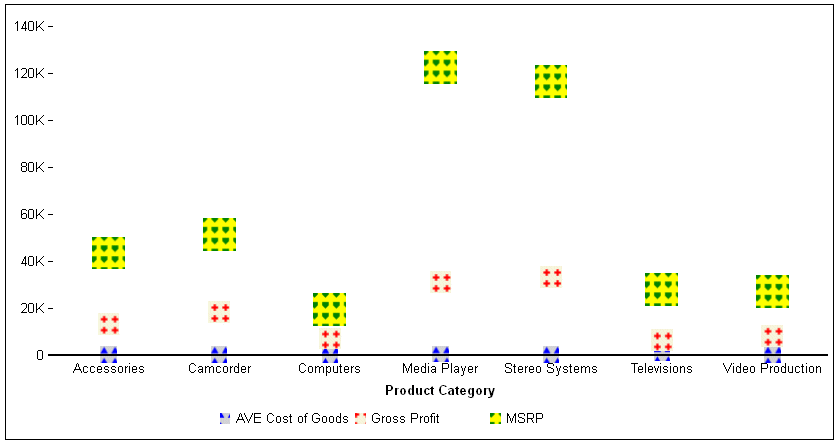WebFOCUS